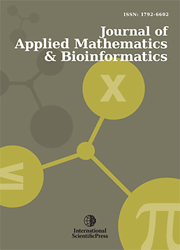# Journal of Applied Mathematics & Bioinformatics

#### A comparative study of a class of implicit multi- derivative methods for the numerical solution of second-order ordinary differential equations

•[ Download ]
• Times downloaded: 927
• Abstract

In this paper, we considered the development, analysis and implementation of a class of multi-derivative methods for solving second order ordinary differential equations. These multi derivatives methods incorporated more analytical properties of the differential equations into the conventional implicit linear multistep method. The step -size (k) and the order of the derivatives (l) methods have been varied to ensure accuracy and efficiency in the methods. The basic properties of these methods were analyzed and the results show that methods are accurate, consistent and zero stable. Comparative studies of the methods were carried out to determine the effect of increasing the step size (k) and the order of the derivatives (l) using some second order ODES. The result shows that the methods are more efficient and accurate when the step-size (k) and order of derivative are increased.ISSN: 1792-6939 (Online)
1792-6602 (Print)